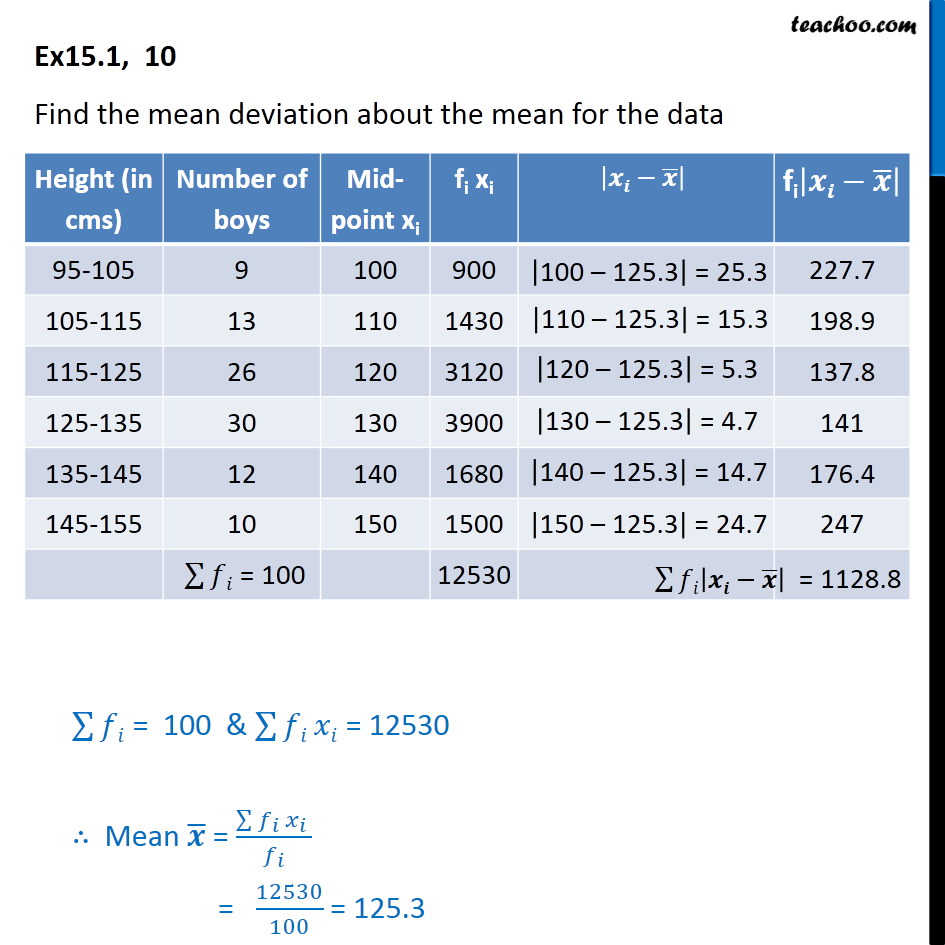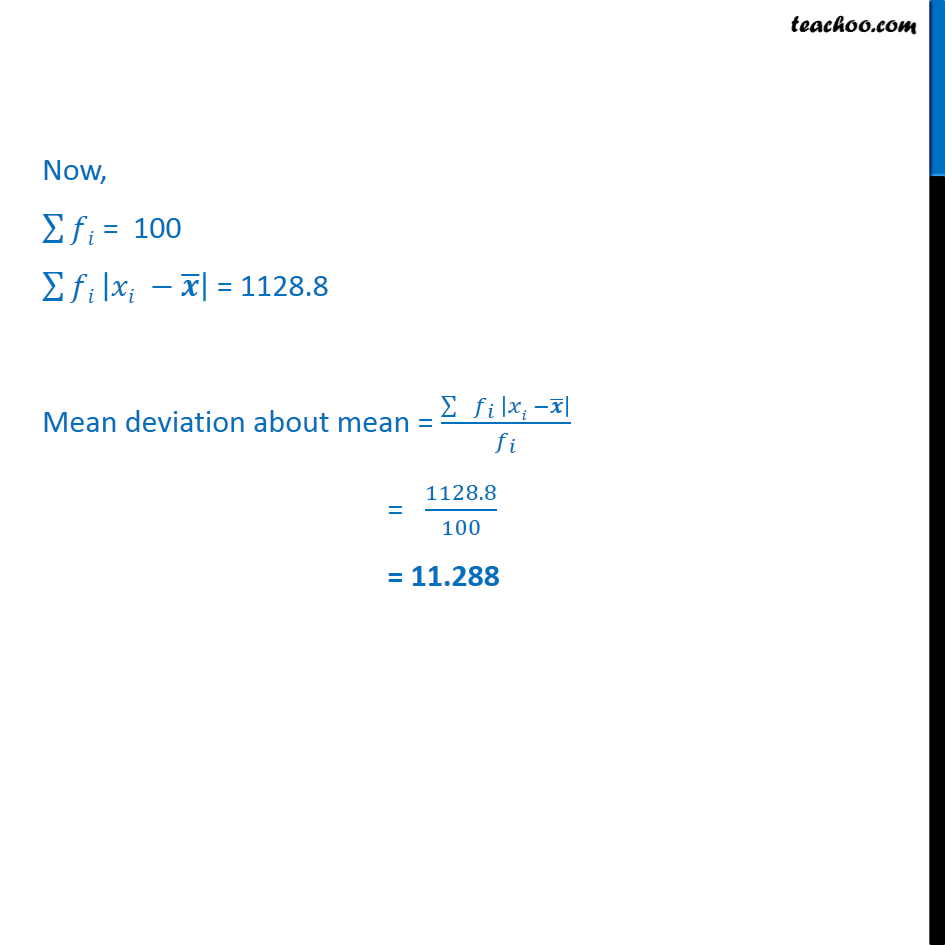1. Chapter 15 Class 11 Statistics
2. Serial order wise
3. Ex 15.1

Transcript

Ex 15.1, 10 Find the mean deviation about the mean for the data Now, ∑128▒𝑓𝑖 = 100 ∑128▒𝑓𝑖 |𝑥𝑖 −𝒙 ̅ | = 1128.8 Mean deviation about mean = (∑▒〖 𝑓_𝑖 |𝑥𝑖 −𝒙 ̅ | 〗)/𝑓_𝑖 = 1128.8/100 = 11.288

Ex 15.1

Chapter 15 Class 11 Statistics
Serial order wise